# [原创]黄金比例搜索算法（Golden Section Search）的实现

<定义>数学上，集合 S 的内部（又称开核）含有所有直观上“不在 S 的边界上”的 S 的点。S 的内部中的点称为 S 的内点。

（1） $f(c) \le f(d)$ ，则极小值出现在子区间 $[a,d]$ 中，所以我们用d代替b，继续在子区间 $[a,d]$ 中搜索；

（2） $f(d) < f(c)$ ，则极小值出现在子区间 $[c,b]$ 中，所以我们用c代替a，继续在子区间 $[a,c]$ 中搜索。

（3）c=a+(1-r)(b-a)=ra+(1-r)b

（4）d=b-(1-r)(b-a)=(1-r)a+rb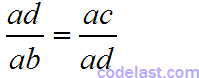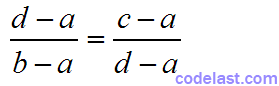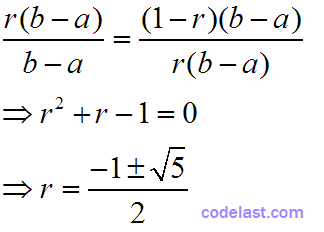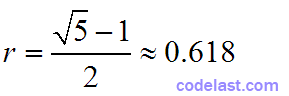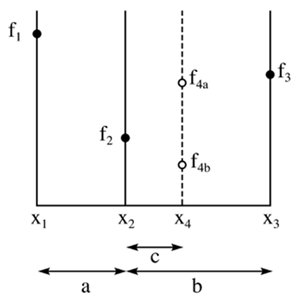${x_1},{x_2},{x_3}$ 是已知的三个点（函数值“高→低→高”），它们划界了一个单峰区间，函数值分别为 ${f_1},{f_2},{f_3}$ 。现在的问题是：如何通过一个新点 ${x_4}$ ，不断缩小该区间的范围，从而逼近极小值点？

${x_4}$ 的函数值为图中 ${f_{4a}}$ 所示时（即 ${f_{4a}} > {f_2}$ ），此时，区间缩小为 ${x_1},{x_2},{x_4}$ （函数值“高→低→高”的3个点）；当 ${x_4}$ 的函数值为图中 ${f_{4b}}$ 所示时（即 ${f_{4b}} < {f_2}$ ），此时，区间缩小为 ${x_2},{x_4},{x_3}$ （函数值“高→低→高”的3个点），每次迭代均按此规则进行选取。

$|{x_3} - {x_1}| < \tau \cdot |{x_2}| + |{x_4}|$

$|{x_3} - {x_1}| < \tau \cdot INIT$$INIT$ 为初始区间长度）

$n \ge \frac{{\lg \delta }}{{\lg 0.618}} + 1$ 取整数。

  public static double PHI = (1 + Math.sqrt(5)) / 2;    // 0.618...
public static double RESPHI = 2 - PHI;  // 0.382...

/**
* Golden section search.
*
* @author Darran Zhang @ codelast.com
* @param x1  The left bound of current bounds.
* @param x2  An interior point between (x1, x3).
* @param x3  The right bound of current bounds.
* @param tau The error tolerance to judge the convergence, recommended value is e^0.5, where e is is the required absolute precision of f(x).
*/
public double goldenSectionSearch(double x1, double x2, double x3, double tau) {
double x4;
if (x3 - x2 > x2 - x1) {    // b > a，右半区间长度较大
x4 = x2 + RESPHI * (x3 - x2);   // x4安插在右半区间
} else {    // b <= a，左半区间长度较大
x4 = x2 - RESPHI * (x2 - x1);   // x4安插在左半区间
}
if (Math.abs(x3 - x1) < tau * (Math.abs(x2) + Math.abs(x4))) {    // 判断是否达到收敛条件
return (x3 + x1) / 2;   // 达到收敛条件，取区间长度的一半作为极小值点输出
}

assert (function.func(x4) != function.func(x2));

/* 未达到收敛条件，继续搜索 */
if (function.func(x4) < function.func(x2)) {    // x4点的函数值如图中f4b所示时
if (x3 - x2 > x2 - x1) {    // b > a，右半区间长度较大
return goldenSectionSearch(x2, x4, x3, tau);  // 以x2，x4，x3作为新三点，继续搜索
} else {    // b <= a，左半区间长度较大
return goldenSectionSearch(x1, x4, x2, tau);  // 以x1，x4，x2作为新三点，继续搜索
}
} else {    // x4点的函数值如图中f4a所示时
if (x3 - x2 > x2 - x1) {    // b > a，右半区间长度较大
return goldenSectionSearch(x1, x2, x4, tau);  // 以x1，x2，x4作为新三点，继续搜索
} else {  // b <= a，左半区间长度较大
return goldenSectionSearch(x4, x2, x3, tau);  // 以x4，x2，x3作为新三点，继续搜索
}
}
}

➤➤ 版权声明 ➤➤### 《[原创]黄金比例搜索算法（Golden Section Search）的实现》有4条评论

1. 文中

（2） f(d)<f(c)，则极小值出现在子区间 [c,b] 中，所以我们用c代替a，继续在子区间 [a,c] 中搜索;

应该是

继续在 [c, b] 中搜索吧...

回复
2. 计算新的函数的结果满足什么条件才终止寻找点？

回复